# Performance Analysis of a Self-excited Induction Generator Mathematical Dynamic Model with Magnetic Saturation, Cross Saturation Effect and Iron Losses

Performance Analysis of a Self-excited Induction Generator Mathematical Dynamic Model with Magnetic Saturation, Cross Saturation Effect and Iron Losses
Ecole Supérieure des Sciences Appliquées d’Alger, Département Second Cycle, Alger 16000, Algeria

Laboratoire de Technologie Industrielle et de l’Information (LTII), Faculté de Technologie, Université de Bejaia 06000, Algeria

Laboratoire d’Electrotechnique et d’Electronique de puissance (L2EP), Université de Lille 1, 59655, France

Corresponding Author Email:
a.aberbour@g.essa-alger.dz
Page:
527-538
|
DOI:
https://doi.org/10.18280/mmep.070404
2 October 2020
|
Accepted:
4 December 2020
|
Published:
18 December 2020
| Citation

OPEN ACCESS

Abstract:

Construction of appropriate mathematical model of plant (object to be modeled) has especially important meaning for designing controller. A reliable and good control performance requires more detailed model. Nevertheless, the latter must be established from at least two viewpoints of preciseness and compactness. In this paper, the considered plant is a self-excited induction generator (SEIG). In order to improve the accuracy, we take into consideration in the SEIG modeling: magnetic saturation phenomenon, cross saturation effect and iron losses. To our best knowledge, this is the first time that the three mentioned phenomena have been integrated into the same and single SEIG model which is presented in an inherent mathematical form. As we will see in the results, the contribution of these three phenomena in the accuracy improvement is really significant. In addition, to achieve compactness of the proposed model, a simpler configuration is obtained for the electrical equivalent circuit associated to the studied model by using Thevenin transformation. The proposed model is built in MATLAB-SIMULINK environment and used to study and analyze the performance of a SEIG under various operating point conditions. The obtained results are compared to measurements and also to the values obtained from models without the three discussed phenomena.

Keywords:

analytical model, induction generator, self-excitation, magnetic saturation, cross saturation effect, iron losses

1. Introduction

The development of simple and accurate analytical models to study the dynamic behavior of induction machines, either in motor or generator operating, has generated a lot of work for several years. The simplest model includes only constant-valued parameters. Such a model leads to relatively accurate results when the operating point studied is around the conditions of the model parameter identification; i.e. motor operating at rated voltage. However, it is well known that the air gap of induction machines is generally narrow, which leads to a non negligible saturation effect, i.e. the inductance values decrease as the flux increases. Therefore, the nonlinearity of the magnetic materials has to be taken into account in the machine model in order to improve the accuracy of simulation studies for a wide range of magnetic states. This condition is even necessary to study the voltage build up of an isolated induction generator, since the linear model is not able to describe the behavior of the system [1-3]. In this aim, more advanced machine models have been proposed. Commonly, the saturation phenomenon is modeled by adjusting the magnetizing inductance as a function of the magnetizing current, or as a function of the flux linkages, using different approaches such as power function [4, 5] or saturation degree function [6, 7]. But these methods need the knowledge of the linear and saturated components of the magnetizing flux, which complicates the identification procedure. A simple and accurate method consists in using polynomial approximation based on experimental data  which can also take into account the cross saturation effect.

The accuracy of the model prediction can also be improved by including the effect of the iron losses which depend both on the magnetizing flux and frequency. Many works dealt with the study, calculation or identification of iron losses [8-12] and induction machine parameters [13-16]. In reference , a model which includes iron losses via an equivalent iron loss resistance is presented. However, the latter is considered constant which can cause detuning of torque and flux in the corresponding vector control system as reported by Levi et al. . Ranta et al.  proposed a model in which iron losses are divided into two components, hysteresis and eddy current losses. The first component is proportional to the frequency while the second one is proportional to the square of the frequency. The dynamic model of a self excited induction generator (SEIG) presented by Bašić et al.  takes the iron losses into account through a non-linear resistance which depends on two dimensional parameters (magnetizing flux and synchronous frequency). Derived from the equivalent electrical circuit with the iron loss resistance placed in parallel to the magnetizing inductance, this model gives an accurate describing of the SEIG behavior. But, it leads to 2nd order differential equations which complicates the corresponding control design and implies high computational needs.

The model presented by Shinnaka  includes iron loss resistance connected in parallel to the stator inductance. This model supposes that the stator current is decomposed into two parts; iron loss current which represents purely stator iron losses and load current which is linked to the flux and torque generation. Such a representation leads to a relatively accurate model when compared to conventional model neglecting iron losses and also facilitates control design compared to the model with an iron loss resistance placed in parallel to the magnetizing inductance. Furthermore, it can be simplified resulting in an equivalent electrical circuit similar to the conventional one, i.e. without iron losses. This approach has been adopted by Bašić et al.  to model a SEIG system but without accounting for the cross saturation effect. The latter can be considered as a magnetic coupling between the windings of the diphase model, and it is reported that the cross saturation effect plays a significant role during the transient state of the electrical variables , and enable a very accurate prediction of the machine’s performance, especially on the voltage build-up of SEIG.

In the present paper, an analytical model which combines simplicity and accuracy and aimed to the study of SEIG is proposed and analyzed. The proposed approach represents a coupling between two previously introduced approaches [1, 2]. The model presented by Idjdarene et al.  takes into consideration magnetic saturation and cross saturation effect but iron losses are omitted. While, the model presented by Bašić et al.  includes magnetic saturation phenomenon and iron losses but the cross saturation effect is neglected. So, we take the initiative to contribute to the accuracy improvement of the SEIG model prediction by including the three mentioned phenomena into account in the same and single model. As we will see below in this article, the inclusion of the magnetic saturation phenomenon is a crucial condition in the SEIG modeling. Cross saturation effect acts in the transient state and intervenes in the improvement of the SEIG voltage build-up. While, integration of iron losses intervenes in the accuracy improvement of the rotor electrical variables and electromagnetic torque. Concerning the methodology adopted in the construction of the proposed model; we used the mathematical model of Idjdarene et al.  (which includes only magnetic saturation and cross saturation effect) as a starting point. Then, we took advantage of the approach adopted by Bašić et al.  to include iron losses and also to obtain a more compact configuration of the electrical equivalent circuit of the induction machine (which operates as a generator) by using the Thevenin transformation. Finally, we end up with a mathematical model which combines simplicity and precision with inherent equations. Still, the SEIG model can gains more refinement by adding the rotor resistance variations with respect to temperature and also by including the skin effect of the rotor bars, but this is not within the scope of this paper.

In the proposed model, the saturation effect and cross saturation effect are taken into account through a variable magnetizing inductance with respects to the magnetizing current while iron losses are modeled via a variable equivalent iron loss resistance with respect to two dimensional parameters (magnetizing flux and stator frequency). To validate the proposed model, the values of both components depending on the above variables are first identified experimentally for a 5.5 kW squirrel cage induction test machine. Then, using MATLAB®-SIMULINK® package, simulations are carried out to study the behavior of the test machine as a SEIG system for two different values of the capacitive bank (100μF and 120μF) under a wide speed range and different load states. The obtained results are compared to measurements for the same operating points. They are also compared to those obtained using conventional model, i.e. without iron losses and cross saturation effect, in order to highlight the accuracy of the proposed model.

2. Mathematical Model of the Induction Machine

The mathematical model that we seek to obtain after development can be considered as an extension of that developed by Idjdarene et al. . So, we will use the induction machine dynamic model of Idjdarene et al.  as the starting point. Using the Clarke transform, the rotor and stator electric equations, which are expressed in stationary reference frame, are given by Eq. (1):

$\left\{ \begin{matrix} {{V}_{s\alpha }}={{R}_{s}}{{i}_{s\alpha }}+{{l}_{s}}\frac{d{{i}_{s\alpha }}}{dt}+{{L}_{m\alpha }}\frac{d{{i}_{m\alpha }}}{dt}+{{L}_{\alpha \beta }}\frac{d{{i}_{m\beta }}}{dt}\begin{matrix} {} & {} & \begin{matrix} {} & \begin{matrix} \begin{matrix} {} & {} \\\end{matrix} & \begin{matrix} {} & \begin{matrix} \begin{matrix} \begin{matrix} {} & {} \\\end{matrix} & {} \\\end{matrix} & {} & {} \\\end{matrix} \\\end{matrix} \\\end{matrix} \\\end{matrix} \\\end{matrix} \\ {{V}_{s\beta }}={{R}_{s}}{{i}_{s\beta }}+{{l}_{s}}\frac{d{{i}_{s\beta }}}{dt}+{{L}_{\alpha \beta }}\frac{d{{i}_{m\alpha }}}{dt}+{{L}_{m\beta }}\frac{d{{i}_{m\beta }}}{dt}\begin{matrix} {} & {} & \begin{matrix} \begin{matrix} \begin{matrix} \begin{matrix} \begin{matrix} \begin{matrix} {} & {} \\\end{matrix} & {} \\\end{matrix} & {} \\\end{matrix} & {} \\\end{matrix} & {} & {} \\\end{matrix} & \begin{matrix} {} & {} \\\end{matrix} \\\end{matrix} \\\end{matrix} \\ 0=-{{R}_{r}}{{i}_{s\alpha }}+{{\omega }_{r}}{{l}_{r}}{{i}_{s\beta }}+{{R}_{r}}{{i}_{m\alpha }}-{{\omega }_{r}}\left( {{l}_{r}}+{{L}_{m}} \right){{i}_{m\beta }}-{{l}_{r}}\frac{d{{i}_{s\alpha }}}{dt}+\left( {{l}_{r}}+{{L}_{m\alpha }} \right)\frac{d{{i}_{m\alpha }}}{dt}+{{L}_{\alpha \beta }}\frac{d{{i}_{m\beta }}}{dt} \\ 0=-{{R}_{r}}{{i}_{s\beta }}-{{\omega }_{r}}{{l}_{r}}{{i}_{s\alpha }}+{{R}_{r}}{{i}_{m\beta }}+{{\omega }_{r}}\left( {{l}_{r}}+{{L}_{m}} \right){{i}_{m\alpha }}-{{l}_{r}}\frac{d{{i}_{s\beta }}}{dt}+{{L}_{\alpha \beta }}\frac{d{{i}_{m\alpha }}}{dt}+\left( {{l}_{r}}+{{L}_{m\beta }} \right)\frac{d{{i}_{m\beta }}}{dt} \\\end{matrix} \right.$     (1)

where, Rs, ls, Rr and lr are the stator and rotor phase resistances and leakage inductances respectively and wr is the rotor electrical pulse. Besides, V, i, V and i are the α-β stator voltages and currents respectively and i and i represent the magnetizing currents, along the α and β axis, such as:

$\left\{ \begin{matrix} {{i}_{m\alpha }}\,=\,{{i}_{s\alpha }}\,+\,{{i}_{r\alpha }} \\ {{i}_{m\beta }}\,=\,{{i}_{s\beta }}\,+\,{{i}_{r\beta }} \\\end{matrix} \right.$     (2)

where, i and i are the rotor current components.

In the model of Idjdarene et al. , only magnetic saturation phenomenon and cross saturation effect are included, respectively through the magnetizing inductance Lm and its derivative Lm’ as a function of the magnetising current im:

${{i}_{m}}\,=\,\sqrt{{{i}_{m\alpha }}_{{}}^{2}\,+\,{{i}_{m\beta }}_{{}}^{2}}$     (3)

Tus, the other inductances introduced in the Eq. (1), Lmα, Lmβ and Lαβ can be expressed as follows:

\left\{ \begin{matrix} {{L}_{m\alpha }}={{L}_{m}}+{{L}_{m}}_{{}}^{'}.\frac{{{i}_{m\alpha }}_{{}}^{2}}{\left| {{i}_{m}} \right|} \\ \begin{align} & {{L}_{m\beta }}={{L}_{m}}+{{L}_{m}}_{{}}^{'}.\frac{{{i}_{m\beta }}_{{}}^{2}}{\left| {{i}_{m}} \right|} \\ & {{L}_{\alpha \beta }}={{L}_{m}}_{{}}^{'}.{{\frac{{{i}_{m\alpha }}.{{i}_{m\beta }}}{\left| {{i}_{m}} \right|}}_{\begin{matrix} {} & \begin{matrix} {} & {} \\\end{matrix} \\\end{matrix}}} \\\end{align} \\\end{matrix} \right.     (4)

where, Lm’ is the derivative of the magnetizing inductance with respect to the magnetizing current im.

The mathematical model introduced above can be illustrated by the equivalent electrical circuit shown in Figure 1 where the iron losses are neglected.

This model is widely used to study machine behaviors or to design control strategies. However, to reach more accurate and efficient torque response, iron losses cannot be neglected but this can lead to a more complex model. In the following, we introduce an analytical approach which constitutes an improvement of the above presented model by introducing a resistance Rf, parallel to ls+Lm, that accounts for iron losses as shown in Figure 2(a).

## 1.png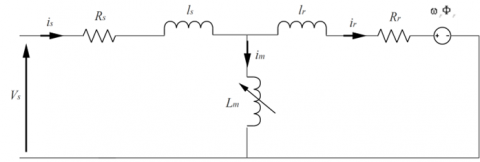Figure 1. Equivalent electrical circuit without iron losses

## 2.pngFigure 2. Equivalent electrical circuit with iron losses, (a) model with Rf parallel to ls+Lm, (b) thevenin equivalent model

Thus, the stator current is can be decomposed into two components; iron loss current iRf that represents purely stator iron losses and load current iL which is linked to the flux and torque generation. From the scheme shown in Figure 2(a), a simpler configuration can be obtained by transforming the “stator loss part” leading to the equivalent electrical circuit shown in Figure 2(b). VsT, isT, RsT are the thevenin variables whose relations are expressed in the diphase α-β frame as follows:

${{R}_{sT}}=\frac{{{R}_{s}}{{R}_{f}}}{{{R}_{s}}+{{R}_{f}}}$     (5)

\left\{ \begin{align} & {{V}_{sT\alpha }}={{V}_{s\alpha }}\frac{{{R}_{f}}}{{{R}_{s}}+{{R}_{f}}} \\ & {{V}_{sT\beta }}={{V}_{s\beta }}\frac{{{R}_{f}}}{{{R}_{s}}+{{R}_{f}}} \\\end{align} \right.     (6)

\left\{ \begin{align} & {{i}_{sT\alpha }}={{i}_{s\alpha }}\frac{{{R}_{s}}+{{R}_{f}}}{{{R}_{f}}}-\frac{{{V}_{s\alpha }}}{{{R}_{f}}} \\ & {{i}_{sT\beta }}={{i}_{s\beta }}\frac{{{R}_{s}}+{{R}_{f}}}{{{R}_{f}}}-\frac{{{V}_{s\beta }}}{{{R}_{f}}} \\\end{align} \right.     (7)

We can note that the equivalent electrical circuit models shown in Figure 1 and Figure 2(b) are similar. Thus, the mathematical equations of the conventional IM model introduced previously, i.e. Eq. (1) to Eq. (4), remain valid for the Thevenin equivalent model including the iron losses by considering the following substitutions:

1. Rs by RsT ;
2. isα by isTα and isβ by isTβ;
3. Vsα by VsTα and Vsβ by VsTβ;

Therefore, the non-linear analytical model of the IM that includes both magnetic saturation and iron losses phenomenon can be expressed as follows:

$\left\{ \begin{matrix} {{V}_{sT\alpha }}={{R}_{sT}}{{i}_{sT\alpha }}+{{l}_{s}}\frac{d{{i}_{sT\alpha }}}{dt}+{{L}_{m\alpha }}\frac{d{{i}_{m\alpha }}}{dt}+{{L}_{\alpha \beta }}\frac{d{{i}_{m\beta }}}{dt}\begin{matrix} {} & {} & \begin{matrix} {} & \begin{matrix} \begin{matrix} {} & {} \\\end{matrix} & \begin{matrix} {} & \begin{matrix} \begin{matrix} \begin{matrix} {} & {} \\\end{matrix} & {} \\\end{matrix} & {} & {} \\\end{matrix} \\\end{matrix} \\\end{matrix} \\\end{matrix} \\\end{matrix} \\ {{V}_{sT\beta }}={{R}_{sT}}{{i}_{sT\beta }}+{{l}_{s}}\frac{d{{i}_{sT\beta }}}{dt}+{{L}_{\alpha \beta }}\frac{d{{i}_{m\alpha }}}{dt}+{{L}_{m\beta }}\frac{d{{i}_{m\beta }}}{dt}\begin{matrix} {} & {} & \begin{matrix} \begin{matrix} \begin{matrix} \begin{matrix} \begin{matrix} \begin{matrix} {} & {} \\\end{matrix} & {} \\\end{matrix} & {} \\\end{matrix} & {} \\\end{matrix} & {} & {} \\\end{matrix} & \begin{matrix} {} & {} \\\end{matrix} \\\end{matrix} \\\end{matrix} \\ 0=-{{R}_{r}}{{i}_{sT\alpha }}+{{\omega }_{r}}{{l}_{r}}{{i}_{sT\beta }}+{{R}_{r}}{{i}_{m\alpha }}-{{\omega }_{r}}\left( {{l}_{r}}+{{L}_{m}} \right){{i}_{m\beta }}-{{l}_{r}}\frac{d{{i}_{sT\alpha }}}{dt}+\left( {{l}_{r}}+{{L}_{m\alpha }} \right)\frac{d{{i}_{m\alpha }}}{dt}+{{L}_{\alpha \beta }}\frac{d{{i}_{m\beta }}}{dt} \\ 0=-{{R}_{r}}{{i}_{sT\beta }}-{{\omega }_{r}}{{l}_{r}}{{i}_{sT\alpha }}+{{R}_{r}}{{i}_{m\beta }}+{{\omega }_{r}}\left( {{l}_{r}}+{{L}_{m}} \right){{i}_{m\alpha }}-{{l}_{r}}\frac{d{{i}_{sT\beta }}}{dt}+{{L}_{\alpha \beta }}\frac{d{{i}_{m\beta }}}{dt}+\left( {{l}_{r}}+{{L}_{m\beta }} \right)\frac{d{{i}_{m\beta }}}{dt} \\\end{matrix} \right.$        (8)

Besides, the flux, magnetizing current components and electromagnetic torque equations can be written, in the diphase frame, under the following forms:

$\left\{ \begin{matrix} {{i}_{m\alpha }}\,=\,{{i}_{sT\alpha }}\,+\,{{i}_{r\alpha }} \\ {{i}_{m\beta }}\,=\,{{i}_{sT\beta }}\,+\,{{i}_{r\beta }} \\\end{matrix} \right.$     (9)

$\left\{ \begin{matrix} {{\Phi }_{s\alpha }}={{L}_{s}}{{i}_{sT\alpha }}+{{L}_{m}}{{i}_{r\alpha }} \\ {{\Phi }_{s\beta }}={{L}_{s}}{{i}_{sT\beta }}+{{L}_{m}}{{i}_{r\beta }} \\ {{\Phi }_{r\alpha }}={{L}_{r}}{{i}_{r\alpha }}+{{L}_{m}}{{i}_{sT\alpha }} \\ {{\Phi }_{r\beta }}={{L}_{r}}{{i}_{r\beta }}+{{L}_{m}}{{i}_{sT\beta }} \\\end{matrix} \right.$     (10)

${{T}_{em}}=\frac{3}{2}p\frac{{{L}_{m}}}{{{L}_{r}}}\left( {{\Phi }_{r\alpha }}{{i}_{sT\beta }}-{{\Phi }_{r\beta }}{{i}_{sT\alpha }} \right)$     (11)

where, Ф, Ф and Ф, Ф are the α and β-axis stator and rotor flux components respectively. Ls= ls+Lm and Lr=lr +Lm are the stator and rotor self inductances, p is the machine pole pair number.

The model developed above can be used to study the behavior of the machine either in motor or generator operating. However, as the effects of saturation phenomenon, cross saturation effect and iron losses are more crucial in generator operating, we use it to study the behavior of a SEIG system whose accurate results are mainly dependant of the model.

3. Magnetic Saturation and Iron Losses

This section deals with the extraction of the non-linear behavior of both magnetic material and stator iron losses of a 5.5kW squirrel cage induction test machine whose rated values are given in appendix (1). A Digital wattmeter and a programmable AC supply which can deliver a voltage with variable magnitude and frequency are used for this purpose, see Figure 3.

We identified the IM iron losses and magnetizing curve experimentaly at no load testing for différent voltage and frequency values. Then, we used method described by Bašić et al.  and Boldea et al.  to calculate the iron loss resistance ans also the magnetizing inductance based on the electrical equivalent circuit of Figure 2(a).

## 3.png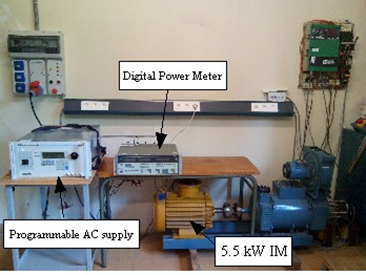Figure 3. Experimental bench

3.1 Magnetic saturation and cross saturation effect

From experimental measures, the values of the magnetizing inductance are identified for different frequencies and magnetizing current magnitudes as shown in Figure 4.

As expected, Lm is frequency independent. Thus, the magnetic saturation is modeled by expressing the magnetizing inductance with respects to the magnetizing current im. To do that, we use the simplest way, i.e. a polynomial approximation.

$\left\{ \begin{matrix} {{L}_{m}}\,=\,f\left( \left| i_{m}^{{}} \right| \right)\,=\,\sum\limits_{j=0}^{n}{a_{j}^{{}}\,.\,\left| i_{m}^{{}} \right|_{{}}^{j}}\begin{matrix} {} & {} & {} \\\end{matrix} \\ {} \\ {{L}_{m}}_{{}}^{'}\,=\frac{d{{L}_{m}}}{d\left| i_{m}^{{}} \right|}\,=\,\frac{d}{d\left| i_{m}^{{}} \right|}f\left( \left| i_{m}^{{}} \right| \right)\,=\,\sum\limits_{j=0}^{n}{j\,.\,a_{j}^{{}}\,.\,\left| i_{m}^{{}} \right|_{{}}^{j-1}} \\\end{matrix} \right.$     (12)

The comparison between measurements and approximated values of Lm(im) shows a very good agreement (Figure 5). In the simulation, the unsaturated part of the characteristic Lm(im) for im<1.2A is approximated with a constant value of 0.105H. This is well justified since the steady state operating point of the SEIG is located in the saturated part, i.e. for im>1.2A. So, this choice will not affect the analysis conducted in this paper.

## 4.png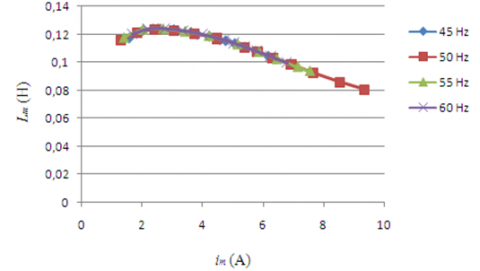Figure 4. Experimental determination of the magnetizing inductance curve

## 5.png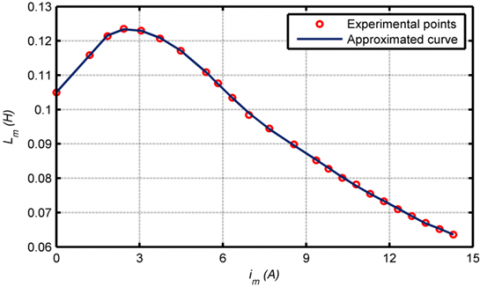Figure 5. Magnetizing inductance versus magnetizing current, obtained by polynomial approximation

Cross saturation effect is taken into account through Lm’ which represents the derivative of the magnetizing inductance with respect to magnetizing current. The evolution of the polynomial derivative of the characteristic Lm(im) with respect to im is shown in Figure 6 below.

## 6.png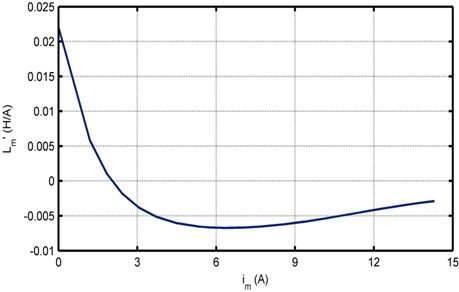Figure 6. Evolution of the magnetizing inductance derivative as a function of the magnetizing current

3.2 Iron losses

Iron losses are usually divided into hysteresis losses and eddy current losses. Hysteresis losses are proportional to the frequency while the eddy current losses are commonly modeled as proportional to the square of the frequency . Iron losses obviously occur both in the stator and the rotor of the induction machine with the major part in the stator due to the stator frequency which is much higher than the slip one. In some works, iron losses are modeled by an equivalent iron loss resistance placed either in parallel to the magnetizing inductance  or in parallel to the stator inductance [2, 12]. Whatever its location, representing iron losses by an equivalent resistance is the simplest method as it leads to only one component to determine. Thus, the obtained model is not bulky and the identification process is quite simple.

The values for Rf obtained from measures carried out on the induction test machine at no load and expressed with respects to the iron loss current iRf, shows that it depends both on iRf, which corresponds to the flux magnitude, and frequency (Figure 7).

Where, iRf is calculated as follow:

${{i}_{Rf}}=\sqrt{{{\left( {{i}_{s\alpha }}-{{i}_{sT\alpha }} \right)}^{2}}+{{\left( {{i}_{s\beta }}-{{i}_{sT\beta }} \right)}^{2}}}$     (13)

## 7.png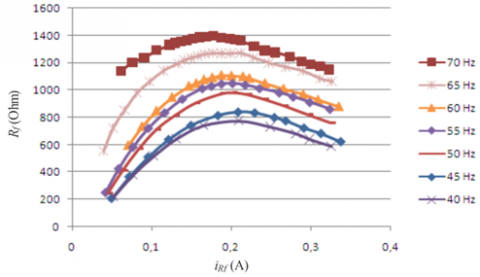Figure 7. Experimental values of the iron loss resistance with respect to iRf and frequency

## 8.png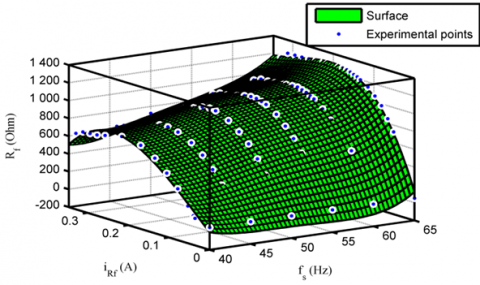Figure 8. Iron loss resistance in function of two variables, iron loss current and frequency

As for the magnetizing inductance, the iron loss resistance characteristic is approximated through a polynomial equation on frequency and on iRf (see appendix). Figure 8 shows the approximated surface along with the measures. The iron loss resistance characteristic Rf(iRf,fs) that is convenient for use in simulation is approximated with a constant value of 50Ω for iRf<0.05A.

The point iRf =0.05A is situated at the left of the iron loss characteristic (Figure 7), which can be characterized as an unstable area where no self-excitation can occur. Therefore, this choice will not affect the analysis conducted in this paper.

4. Model Adaptation to Study a Self-Excited Induction Generator

The system studied is shown in Figure 9. It is constituted of a self excited induction generator whose rotor is connected to a controlled DC motor. The stator windings are star connected to capacitor bank and to three phase resistive load.

## 9.pngFigure 9. Self-excited induction generator system feeding resistive load

The equations related to these parts have to be added to the IM relations to construct the SEIG model. In the case of no load operating, the stator voltages and currents are linked by the following matrix equation:

$\frac{d}{dt}\left[ \begin{matrix} {{V}_{sa}} \\ {{V}_{sb}} \\ {{V}_{sc}} \\\end{matrix} \right]=\left[ \begin{matrix} \frac{1}{C} & 0 & 0 \\ 0 & \frac{1}{C} & 0 \\ 0 & 0 & \frac{1}{C} \\\end{matrix} \right].\left[ \begin{matrix} {{i}_{sa}} \\ {{i}_{sb}} \\ {{i}_{sc}} \\\end{matrix} \right]$     (14)

When the SEIG system is loaded, the load currents ila, ilb and ilc must be added to the above matrix equation that then takes the following form:

$\frac{d}{dt}\left[ \begin{matrix} {{V}_{sa}} \\ {{V}_{sb}} \\ {{V}_{sc}} \\\end{matrix} \right]=\left[ \begin{matrix} \frac{1}{C} & 0 & 0 \\ 0 & \frac{1}{C} & 0 \\ 0 & 0 & \frac{1}{C} \\\end{matrix} \right].\left[ \begin{matrix} {{i}_{sa}}-{{i}_{la}} \\ {{i}_{sb}}-{{i}_{lb}} \\ {{i}_{sc}}-{{i}_{lc}} \\\end{matrix} \right]$     (15)

Finally, in the case of balanced load operating, three identical resistances are considered such as:

$\left\{ \begin{matrix} {{V}_{sa}}={{R}_{l}}{{i}_{la}} \\ {{V}_{sb}}={{R}_{l}}{{i}_{lb}} \\ {{V}_{sc}}={{R}_{l}}{{i}_{lc}} \\\end{matrix} \right.$     (16)

The capacitor-SEIG obtains its magnetizing current from capacitors connected across its output terminals. As the rotor is driven for a certain speed, IM residual magnetization induces a small EMF in the stator windings at a frequency proportional to rotation speed, circulating a current through capacitors. If capacitors are of sufficient value, the voltage builds-up, its final value being limited by the IM magnetic saturation . The choice of the capacitor for self-excitation is very important. The latter must have its value within a certain range to sustain self-excitation. If its value is outside of this range, self excitation will not be possible. The minimum and maximum values of capacitor required for self-excitation of a three-phase induction generator have been analyzed previously [20-23].

In our case, for a given rotation speed, we used the approximate minimum capacitor Cm required for self excitation given by Seyoum  and calculated as:

${{C}_{m}}=\frac{1}{{{\left( p\Omega \right)}^{2}}{{L}_{mn}}}$      (17)

where, Ω in rd/s and Lmn is rated magnetizing inductance calculated at rated magnetizing level which corresponds for the used IM to the value 0.10474H.

However, for economic and technical reasons, the use of excessive capacitor values is not recommended due to the fact that the current can greatly exceed its nominal value . On the other hand, it is not advisable to use the minimum capacitor value either because any change in load or rotor speed may result in loss of excitation. Therefore, we have added a margin of about 10% to 15% with respect to the minimum capacitor value. So, we carried out experiments and simulations for the following speeds and their corresponding capacitor value:

1.  For fixed rotation speeds: 780rpm and 790rpm, required minimum capacitor according to Eq. (17) is respectively 89μF and 87μF. By taking into account an additional margin of 15%, the value of the capacitor used for these two speeds is 100 μF.
2. For fixed rotation speed of 690rpm, the required minimum capacitor according to Eq. (17) is 115μF. With margin of about 10%, we used a capacitor of 120μF.
5. Model Validation

A first step consists in validating the SEIG proposed model through comparisons, at no load, between simulation results and measurements obtained from the experimental bench shown in Figure 10. The latter is made up of the squirrel cage induction test machine mechanically coupled to a driven DC motor. The stator windings of the IM are star connected to a capacitor bank, and eventually, to a three phase variable resistive load.

Different tests were performed for a wide speed range, two values of the capacitor bank (100μF and 120μF) and various resistive loads.

The simulation results are compared to those obtained from the experimental bench and also to the results obtained by models without magnetic saturation, cross saturation effect and iron losses.

## 10.png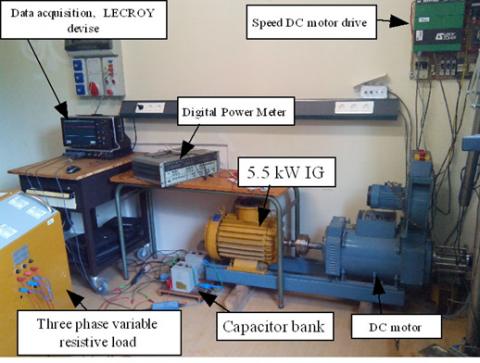Figure 10. Experimental bench of the SEIG system

Note that, a transition from the Thevenin model to the model without iron losses can be obtained by taking an infinite value for Rf. In a practical point of view, Rf should take a very high value . Generaly the space vectors of dynamic model have different angular frequencies in transient states. So in this paper the stator frequency, which is used for the calculation of Rf, is estimated via a PLL through the stator currents. To take account for the remnant EMF, a small value of 5V is assigned as an initial value to the simulated stator voltages for both models.

In the following, we will study case by case the contribution of magnetic saturation, cross saturation effect and iron losses on the model accuracy predictions. Firstly, we will neglect magnetic saturation while including the two other phenomena (Figure 11). Secondly, we will neglect the cross saturation effect while including magnetic saturation and iron losses (Figure 12), and finally, we will neglect iron losses while including magnetic saturation and cross saturation effect (Figure 13).

Figure 11(a) shows the phase voltage build-up obtained when magnetic saturation is neglected i.e. the magnetizing inductance takes a constant value (Lm = Lmn = 0.10474H, which is calculated at rated magnetization of the IM). It is obvious that having a constant magnetizing inductance, the derivative of the magnetizing inductance with respect to magnetizing current, which introduces the cross saturation effect, is automatically canceled (Lm’ = 0H/A).

As observed in Figure 11(a), the induced voltage continues to rise without converging to an equilibrium state. So, the model without magnetic saturation is far from describing the behavior of the real SEIG system whose voltage build-up should be like measure shown in Figure 11(b). Thus, magnetic saturation in SEIG is the main factor in the dynamics of voltage build-up and stabilization, so it must be accounted for in the SEIG model. This statement is confirmed by the results of Figure 12 where magnetic saturation is not neglected, and it is clearly seen that the generated voltage stabilizes and reaches an equilibrium state.

## 11.png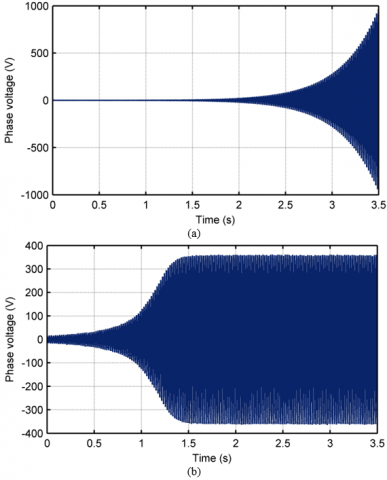Figure 11. Phase voltage built up (100μF at 780rpm), comparison between simulation and measure (a) simulation without magnetic saturation, (b) measure

Figure 12 also conveys information on the interest of taking into account the cross saturation effect. Indeed, we observe a remarkable difference in the transient state of the generated voltages in Figure 12(a) which shows a superposition between the experimental measure and the result obtained with the model which neglects the cross saturation effect. However, in Figure 12(b) which overlaps the experimental measure and result of the full saturated model (with cross saturation effect), extremely good correspondence is revealed. Thus, without a shadow of a doubt, the full saturated SEIG model enables accurate simulation in the voltage build-up. After all, we would like to emphasize that concerning the cross saturation effect, the results obtained by the proposed model favorably agree with the results obtained by Levi , and this even if the models used are different.

## 12.pngFigure 12. Phase voltage built up (100μF at 780rpm), comparison between measure and simulations, (a) without cross saturation, (b) with cross saturation

## 13.png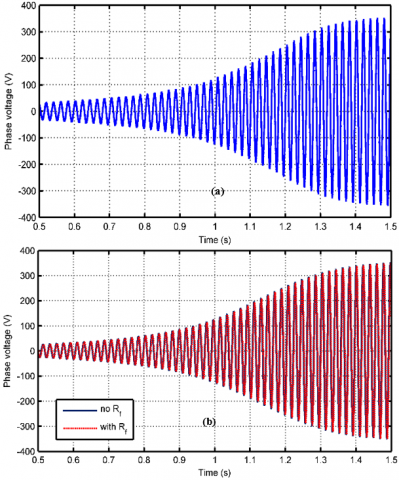Figure 13. Phase voltage built up (100μF at 780rpm), comparison between measure and simulations, (a) measure, (b) Simulation

## 14.pngFigure 14. Zoom of the Phase voltage built up (100μF at 780rpm), comparison between simulations

Figure 13 shows the comparison of the no load voltage built up waveforms obtained by simulation and measurement in the case of a capacitive bank of 100μF and a speed of 780rpm. Figure 13(a) shows the phase voltage obtained by measurement, while Figure 13(b) shows a superposition of phase voltage obtained by the models with and without iron losses. As can be seen, the global waveforms obtained by both models are in good agreement with measurement. However, one can observe from the zoom of Figure 14 that, there is a small time delay as well as a slight difference in the estimation of the phase voltages during the transient.

Figures 15 and 16 show a comparison between measurement and simulation results of the models with and without iron losses for both phase voltage and phase current transient, respectively, when subjected to a step change in load (R= 136Ω) at t=2.5s. These results are obtained in the case of a capacitive bank of 100μF and a speed of 780rpm.

## 15.png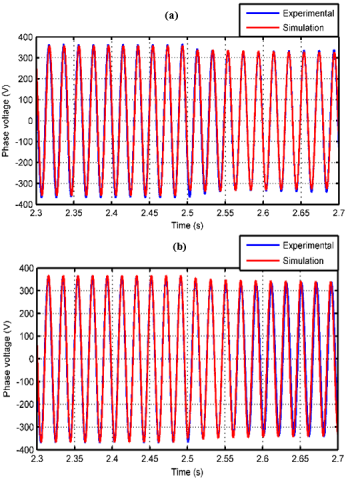Figure 15. Phase voltage transient (100μF at 780rpm), comparison between measure and simulation, (a) with iron losses, (b) without iron losses

From Figure 15(a), the Thevenin model with iron losses is slightly underestimating the magnitude of the phase voltage, while, the model without iron losses, overestimate them (Figure 15(b)).

We also observe a small difference in frequency for t>2.5s in the case of the conventional model, without iron losses, while the proposed model is in good agreement with measurement. The same observation arises from the comparison of the stator currents shown in Figure 16.

Nevertheless, the difference between both models concerning the stator variables predictions is close. But, it will be seen in the following that, the impact of iron losses is much important for the rotor variables predictions and the electromagnetic torque.

## 16.png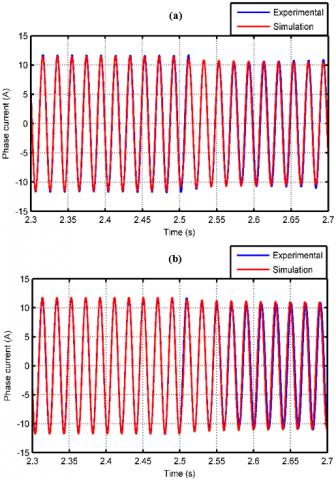Figure 16. Phase current transient (100μF at 780rpm), comparison between measure and simulation, (a) with iron losses, (b) without iron losses

While taking account of the load, various operating points are studied using the proposed model and compared to measures.

For the sake of synthesis, simulations are performed with a resistive load whose value is changed at t=4s (from 385W to 102W) and at t=7s (from 102W to 58W). These three values are similar to some ones used in the experimental tests.

The RMS value of the stator phase voltage and stator current are presented in Figures 17 and 18 respectively where (a) depicts the results obtained at 790rpm and 100μF per phase and (b) the ones of 690rpm and 120μF.

Figure 17 shows a comparison between the RMS value of the stator voltage obtained by both models and measures. The latter are represented by the continuous black line.

As can be seen, the inclusion of iron losses in the IM model induces a slight underestimation of the generated stator voltage magnitude when compared to the results given by the classical model. This difference increases along with the load increase but the results given by both models are very close to the experimental values for all the studied operating points. The same observation arises from the comparison of the stator currents as shown in Figure 18. This difference can be linked to the magnetization level of the machine.

## 17.png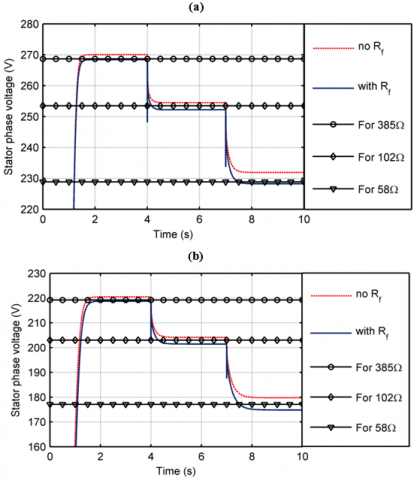Figure 17. RMS value of the stator voltage, comparison between experimental and simulations, (a) results obtained for 100μF at 790rpm, (b) results obtained for 120μF at 690rpm

## 18.png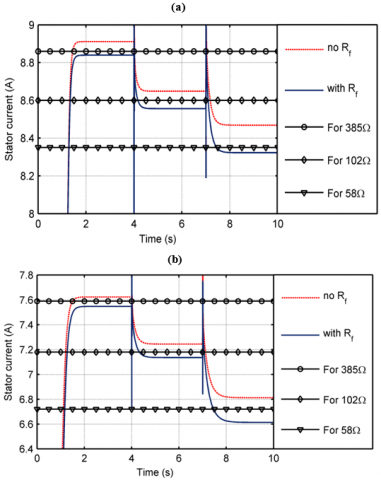Figure 18. RMS value of the stator current, comparison between experimental and simulations, (a) results obtained for 100μF at 790rpm, (b) results obtained for 120μF at 690rpm

Indeed, as can be shown from the waveforms of the magnetizing inductance drawn in Figure 19 for the studied operating points, the classical model leads to a higher saturation level when compared to the results obtained by the model which takes the iron losses into account. This also shows that the difference is more significant in the case of high loads.

## 19.png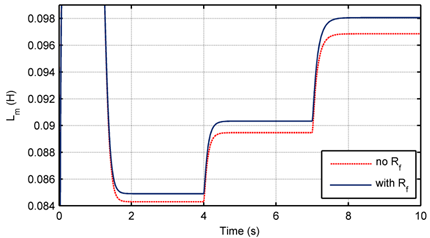Figure 19. Magnetizing inductance, results obtained for 100μF at 790rpm

## 20.png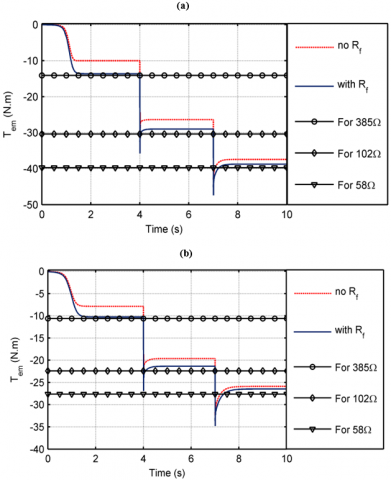Figure 20. Electromagnetic torque, comparison between experimental and simulations, (a) results obtained for 100μF at 790rpm, (b) results obtained for 120μF at 690rpm

Figure 20 shows the electromagnetic torque waveform obtained for the studied operating points. The results from the proposed Thevenin model with iron losses are much more close to measures than the ones given by the model without iron losses. The latter underestimates the electromagnetic torque mainly for light loads, due to the important contribution of iron losses in this case. The values of the electromagnetic torque in Figure 20 obtained by simulations are calculated using Eq. (11) where isTa and isTb are replaced by isa and isb in the case of classical model without iron losses. Then, they are compared to the ones obtained from the experimental bench through the following relation:

$T_{e m}=\frac{P_{\text {load}}-P_{s_{_{-} c o p}}-P_{r_{-} c o p}-P_{\text {iron}}}{\Omega}$     (18)

where, Pload is the measured power supplied to the load, it is a negative value due to the motor convention used in the mathematical model. Ps_cop and Pr_cop are the stator and rotor copper losses respectively and Piron represents the iron losses. The latter is obviously taken equal to zero in the case of the classical model without iron losses. Mechanical losses are not considered as the SEIG is driven by the DC machine. Ω is the rotor rotation speed obtained from the control of the DC drive machine.

Using the equivalent electrical circuit scheme, the rotor currents are calculated for both models. The equivalent experimental value of this current is also deduced from the electromagnetic torque using the well known following relation:

${{i}_{r}}=\sqrt{\frac{s\Omega {{T}_{em}}}{3{{R}_{r}}\left( 1-s \right)}}$     (19)

where, s is the slip value.

Then, the obtained results are compared in Figure 21. As expected from the electromagnetic torque waveforms, the values given by the proposed model are in better agreement with the ones deduced from measures.

## 21.pngFigure 21. Rotor current, comparison between experimental and simulations, (a) results obtained for 100μF at 790rpm, (b) results obtained for 120μF at 690rpm

Finally, from the several operating points simulated and tested, Figure 22 shows the behavior of both iron losses and the global copper losses (stator and rotor copper losses), with respect to electromagnetic torque, obtained by the proposed model and the measurements at 790rpm and 100μF. Both simulated waveforms are in very good agreement with the experimental results. It can be particularly noted that the iron losses are higher than the global copper losses at light loads. We also notice a significant decrease of iron losses with respect to the electromagnetic torque. Given that the rotor speed is kept constant at 790rpm, this can be only due to the significant decrease in magnetizing flux illustrated through the iron loss current iRf shown by the Figure 23.

## 22.pngFigure 22. Iron losses and copper losses versus electromagnetic torque, comparison between experimental and simulation, results obtained for 100μF at 790rpm

## 23.pngFigure 23. Iron loss current versus electromagnetic torque, simulation results obtained for 100μF at 790rpm

The obtained results reflect very well the reality of the IM. Indeed, it is well known that when the IM is driven under light loads at high or rated magnetization level, the flux is higher than necessary to develop the required electromagnetic torque and, due to the unbalance between iron and copper losses; it results in the increase of the IM electrical losses which are referred to stator copper losses, rotor copper losses and iron losses. Moreover, this characteristic of the IM has given rise to a lot of works which seek to minimize IM electrical losses, working in generator or in motor mode, by adapting its magnetization level according to the load level. The higher the flux level is, the larger iron losses are. In the opposite, excessive decrease of the flux level causes higher copper losses. Then, the key to solve the problem of minimizing the IM losses is to obtain the optimum balance between copper and iron losses.

Figure 24 shows the behavior the iron loss resistance Rf with respect to electromagnetic torque. It increases almost linearly with the electromagnetic torque. The Rf behvior is easy to explain: At light loads the generated voltages and currents are higher than when the SEIG operates at high loads (see Figures 17 and 18), which means higher magnetization level and saturation level, and because irf is an image of the magnetization level explains why it is more important at light loads. As the SEIG works in the saturated region of the charactrestic Rf(irf, fs), then Rf takes low values when irf is high. When the load increases, the generated voltages and currents decreases (see Figures 17 and 18) which means the decreas of magnetization level and saturation level, this explain why irf takes low values at high loads, and as the SEIG works at the saturated part of the charactrestic Rf(irf, fs), therefore Rf takes high values. Thus we can say that a high value of the iron loss resistance means iron losses level is low, the reverse is true, low value of iron loss resistance means high level of iron losses. Of course, this statement is valid in the stable operating region of the SEIG (when the IM is saturated), this is natural since no self-excitation is possible in the unsaturated part of the characteristics Rf(irf, fs) and Lm(im).

## 24.png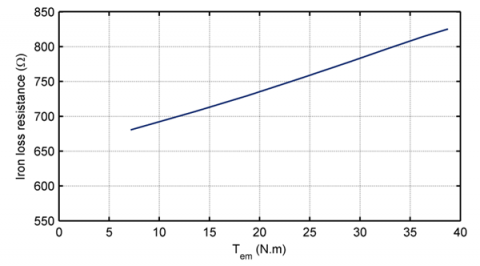Figure 24. Iron loss resistance versus electromagnetic torque, simulation results obtained for 100μF at 790rpm

6. Conclusion

In this paper, a dynamic mathematical model including magnetic saturation, cross saturation effect and iron losses of a SEIG is developped. It is based on the equivalent electrical circuit in which the iron loss resistance is placed in parallel to the stator self inductance. The use of Thevenin transform leads to a compact configuration and a simple mathematical model similar to the well known classical model without iron losses.

Several noticeable results have been obtained in this study through computer simulation and laboratory experiments. They are summarized below:

(1) Magnetic saturation is the key factor in the voltage build-up of SEIG, it is mandatory to include this phenomenon in the SEIG modeling.

(2) The full saturated SEIG model (with magnetic saturation and cross saturation effect) enables very accurate results in the transient of the voltage build-up.

(3) We can put the proposed SEIG model and that one without iron losses at the same level concerning the accuracy prediction of the generated stator voltages and stator currents.

(4) The results obtained by the proposed SEIG model, in terms of rotor current along with electromagnetic torque, are in a better agreement with the measurements than those given by that one without iron losses, more significantly at light loads.

(5) The proposed SEIG model enables very accurate results in the prediction of ion losses and global copper losses. In addition, it reproduces very well the physical reality of the IM about the existence of unbalance between iron losses and global copper losses depending on load level, magnetization level and frequency. So, it can be used as the basis to design loss minimization strategy for SEIG used in autonomous or isolated wind/hydro energy conversion systems where loads and speed can present randomly large variation range.

(6) Due to the compactness of the proposed SEIG model and to its relative simplicity, it can be used to design vector control strategies leading to new outcomes with high-efficiency operating.

Nomenclature
 Vsa stator voltage of phase a, V Vsb stator voltage of phase b, V Vsc stator voltage of phase c, V isa stator current of phase a, A isb stator current of phase b, A isc stator current of phase c, A ila load current of phase a, A ilb load current of phase b, A ilc load current of phase c, A Vsa a-axis stator voltage, V Vsb b-axis stator voltage, V VsTa Thevenin a-axis stator voltage, V VsTb Thevenin b-axis stator voltage, V isa a-axis stator current, A isb b-axis stator current, A isTa Thevenin a-axis stator current, A isTb Thevenin b-axis stator current, A ira a-axis rotor current, A irb b-axis rotor current, A ir rotor current, A iRf iron loss current, A ima a-axis magnetizing current, A imb b-axis magnetizing current, A im magnetizing current, A L ma a-axis magnetizing inductance, H L mb b-axis magnetizing inductance, H Lab cross effect inductance, H L m magnetizing inductance, H L m’ Lmn magnetizing inductance derivative  value of Lm at rated magnetization Ls stator self inductance, H Lr rotor self inductance, H ls stator leakage inductance, H lr rotor leakage inductance, H Rs stator resistance, ohms RsT Thevnin stator resistance, ohms Rr rotor resistance, ohms Rl load resistance, ohms Rf iron loss resistance, ohms C capacitor, F fs stator frequency, Hz p pole pair number Tem electromagnetic torque, Nm Pload measured power supplied to the load, W Ps-cop stator copper losses, W Pr-cop rotor copper losses, W Piron iron losses, W Greek symbols $\Omega$ mechanical rotor speed, rad/s $\omega_{r}$ rotor electrical pulse, rad/s $\Phi_{s \alpha}$ a-axis stator flux, Wb $\Phi_{s \beta}$ b-axis stator flux, Wb $\Phi_{r \alpha}$ a-axis rotor flux, Wb $\Phi_{r \beta}$ b-axis rotor flux, Wb Subscripts SEIG self-excited induction generator IM induction machine
Appendix

(1) Rated parameters of the used IM: PN=5.5kW, UN=230/400V, IN=23.8/13.7A, 50Hz, WN=690rpm, J=0.230kg.m2, d=0.0025N.m/rads-1, Rs=1.07131W, Rr=1.29511W, p=4, Lmn=0.10474H.

(2) Polynomial approximation of the iron loss resistance:

Linear model Poly43:

Sf(x,y) = p00 + p10*x + p01*y + p20*x^2 + p11*x*y + p02*y^2 + p30*x^3 + p21*x^2*y + p12*x*y^2 + p03*y^3 + p40*x^4 + p31*x^3*y + p22*x^2*y^2 + p13*x*y^3

Coefficients:

p00 = 4.269e+004, p10 =-3386, p01= -477.8, p20 =100.4, p11 = 7.263, p02 = 168.8, p30 =-1.322, p21 = 0.3607, p12 =-4.983, p03 = -7.721, p40 = 0.006509, p31 = -0.003933, p22 = 0.02345, p13 = 0.153

References

 Idjdarene, K., Rekioua, D., Rekioua, T., Tounzi, A. (2009). Performance of an isolated induction generator under unbalanced loads. IEEE Transactions on Energy Conversion, 25(2): 303-311. https://doi.org/10.1109/TEC.2009.2036836

 Bašić, M., Vukadinović, D., Petrović, G. (2012). Dynamic and pole-zero analysis of self-excited induction generator using a novel model with iron losses. International Journal of Electrical Power & Energy Systems, 42(1): 105-118. http://dx.doi.org/10.1016/j.ijepes.2012.03.003

 Leplat, P.M., Tounzi, A., Clénet, S., Piriou, F. (1996). Study of an induction machine using Park’s model and finite element method. In Proc. ICEM1996, 2: 24-29. http://hdl.handle.net/20.500.12210/30190

 Qu, Z., Ranta, M., Hinkkanen, M., Luomi, J. (2012). Loss-minimizing flux level control of induction motor drives. IEEE Transactions on Industry Applications, 48(3): 952-961. https://doi.org/10.1109/TIA.2012.2190818

 de Jong, H.C.D. (1980). Saturation in electrical machines. In Proc. ICEM’80, Athens, Greece, 3: 1545-1552.

 Ojo, J.O., Consoli, A., Lipo, T.A. (1990). An improved model of saturated induction machines. IEEE Transactions on Industry Applications, 26(2): 212-221. https://doi.org/10.1109/28.54245

 Ibtiouen, T., Nesba, A., Mekhtoub, S., Touhami, O. (2001). An approach for the modelling of saturated induction machine. In Proc. ACEMP’01, Kasudasi-Turkey, pp. 269-274.

 Leidhold, R., Garcia, G., Valla, M.I. (2002). Field-oriented controlled induction generator with loss minimization. IEEE Transactions on Industrial Electronics, 49(1): 147-156. https://doi.org/10.1109/41.982258

 Levi, E., Sokola, M., Boglietti, A., Pastorelli, M. (1996). Iron loss in rotor-flux-oriented induction machines: Identification, assessment of detuning, and compensation. IEEE Transactions on Power Electronics, 11(5): 698-709. https://doi.org/10.1109/63.535402

 Ranta, M., Hinkkanen, M., Dlala, E., Repo, A.K., Luomi, J. (2009). Inclusion of hysteresis and eddy current losses in dynamic induction machine models. In 2009 IEEE International Electric Machines and Drives Conference, pp. 1387-1392. https://doi.org/10.1109/IEMDC.2009.5075384

 Bašić, M., Vukadinović, D., Lukač, D. (2011). Novel dynamic model of self-excited induction generator with iron losses. International Journal of Mathematical Models and Methods in Applied Sciences, 5(2): 221-229.

 Shinnaka, S. (1998). Proposition of new mathematical models with core loss factor for controlling AC motors. IEEE Proc. Ind. Electronics Society, IECON’98, 1: 297-302. https://doi.org/10.1109/IECON.1998.724139

 Aminu, M., Ainah, P.K., Abana, M., Abu, U.A. (2018). Identification of induction machine parameters using only no-load test measurements. Nigerian Journal of Technology, 37(3): 742-748. http://dx.doi.org/10.4314/njt.v37i3.25

 van den Bosch, P.P.J., van der Klauw, A.C. (1994). Modeling, Identification and Simulation of Dynamical Systems. CRC Press.

 Fathabadi, F.R., Molavi, A. (2019). Black-box identification and validation of an induction motor in an experimental application. European Journal of Electrical Engineering, 21(2): 255-263. https://doi.org/10.18280/ejee.210219

 Toliyat, H.A. Levi, E., Raina, M. (2003). A review of RFO induction motor parameter estimation techniques. IEEE Transactions on Energy Conversion, 18(2): 271-283. https://doi.org/10.1109/TEC.2003.811719

 Levi, E. (1997). Impact of cross-saturation on accuracy of saturated induction machine models. IEEE Transactions on Energy Conversion, 12(3): 211-216. https://doi.org/10.1109/60.629705

 Boldea, I. Nasar, S.A. (2002). The Induction Machine Handbook. CRC Press.

 Nayar, C.V., Perahia, J., Thomas, F., Phillips, S.J., Pryor, T., James, W.L. (1991). Investigation of capacitor-excited induction generators and permanent magnet alternators for small scale wind power generation. Renewable Energy, 1(3-4): 381-388. https://doi.org/10.1016/0960-1481(91)90047-S

 Malik, N.H., Al-Bahrani, A.H. (1990). Influence of the terminal capacitor on the performance characteristics of a self excited induction generator. In IEE Proceedings C, Generation, Transmission and Distribution, 137(2): 168-173. https://doi.org/10.1049/ip-c.1990.0022

 Al Jabri, A.K., Alolah, A.I. (1990). Limits on the performance of the three-phase self-excited induction generators. IEEE Transactions on Energy Conversion, 5(2): 350-356. https://doi.org/10.1109/60.107232

 Wang, L., Lee, C.H. (1997). A novel analysis on the performance of an isolated self-excited induction generator. IEEE Transactions on Energy Conversion, 12(2): 109-117. https://doi.org/10.1109/60.629692

 Harrington, R.J., Bassiouny, F.M.M. (1998). New approach to determine the critical capacitance for self-excited induction generators. IEEE Transactions on Energy Conversion, 13(3): 244-249. https://doi.org/10.1109/60.707603

 Seyoum, D. (2003). The dynamic analysis and control of a self-excited induction generator driven by a wind turbine. Phd. thesis, University of New South Wales, pp. 1-324.